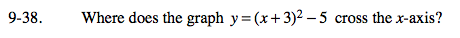Home > A2C > Chapter 9 > Lesson 9.1.2 > Problem9-38

9-38.

Where does the graph y = (x + 3)2 − 5 cross the x-axis? Homework Help ✎Set y equal to 0 and solve for x.

$(-3\pm\sqrt{5},\,0)$